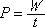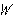power = work divided by time

Power is the rate at which work is done. When an amount of workis done in a time interval from time 0 to time t, the average power during that time interval is given by the formula at the top of this page. If the work is done at a constant rate (also referred to as a steady rate) then the power is a constant and the value calculated by means of this formula is the power appropriate to every instant during the time interval.

 Calculus-Related Note: Power is the derivative of work with respect to time:The work in this expression is the total amount of work done to date as a function of time. Note that if the work is directly proportional to time, the power is a constant and the expression at the top of this page applies for the instantaneous power because the power is a constant.